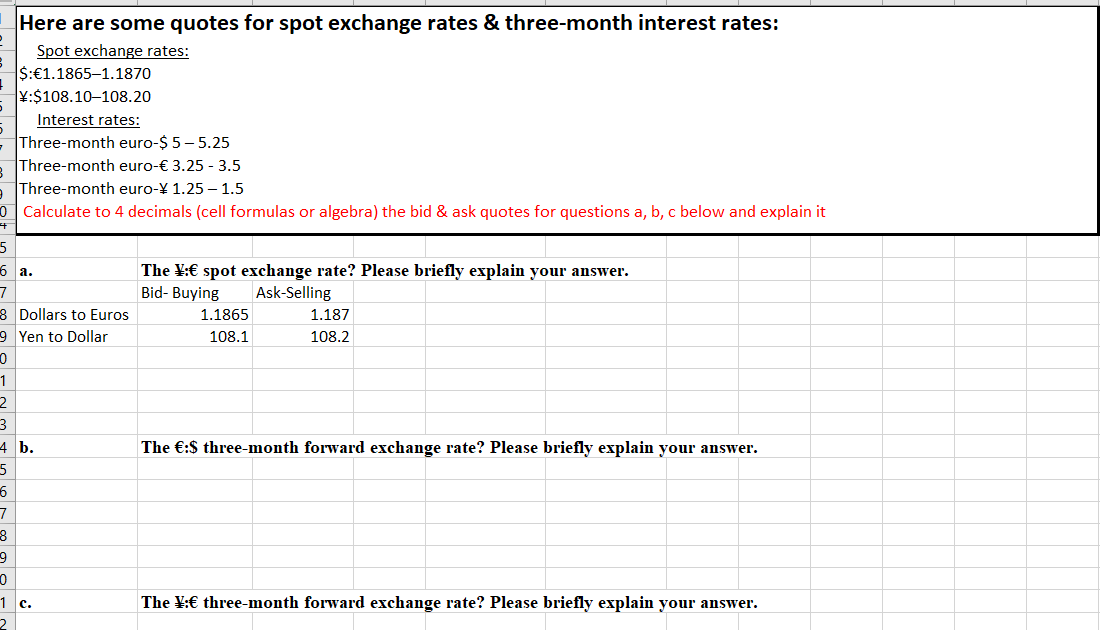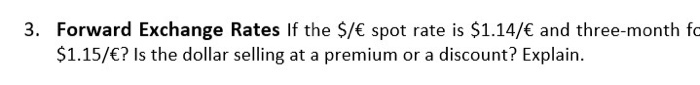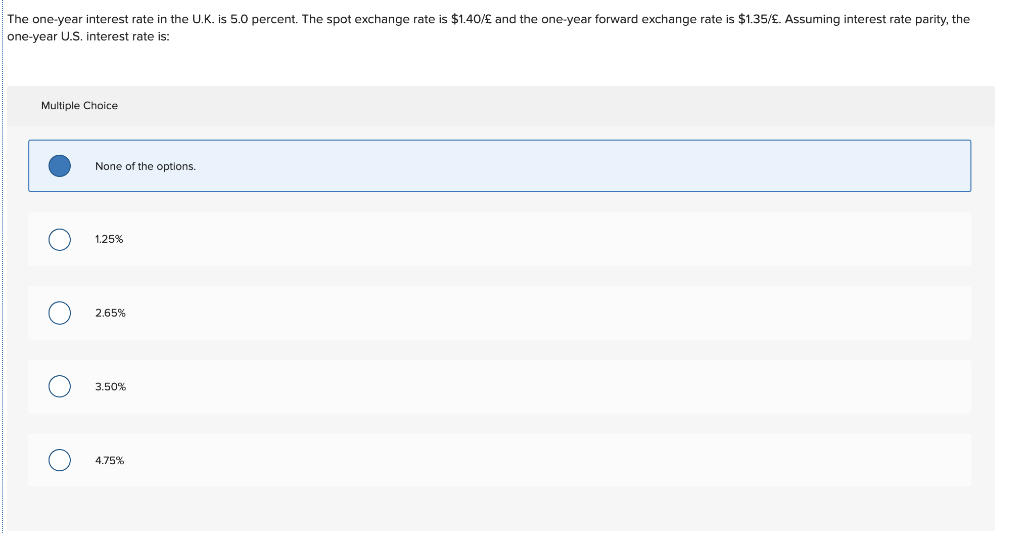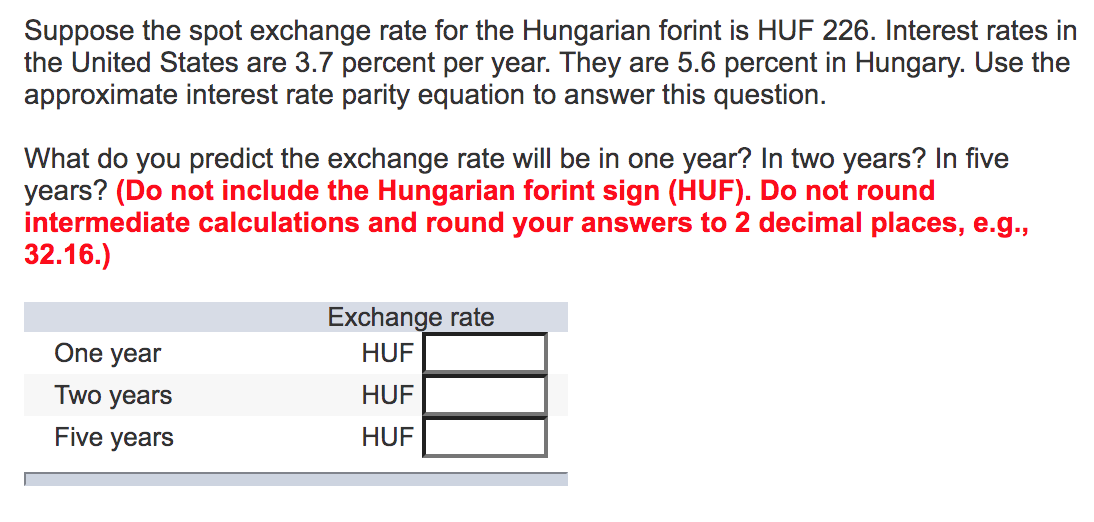Question

# The relationship that links spot exchange rates, interest rates, and forward exchange rates is described as...

The relationship that links spot exchange rates, interest rates, and forward exchange rates is described as the ____________?

A.Interest rate parity theorem

B.Law of one price

D.Foreign exchange

Answer - A. Interest rate parity theorem

Interest rate parity is a theorem that describes interest rate differential between two countries is equal to the differential between the forward exchange rate and the spot exchange rate.

#### Earn Coins

Coins can be redeemed for fabulous gifts.

Similar Homework Help Questions
• ### All interest and inflation rates are stated as annual rates. Unbiased forward rate (forward expectation parity)...

All interest and inflation rates are stated as annual rates. Unbiased forward rate (forward expectation parity) 1. If the spot market exchange rate for the euro is 1.1427 and the 6-month forward quote is 178, what is the expected exchange rate for the euro in six months? 2. If the spot market exchange rate for the Hong Kong dollar is 7.8461 and the 1-year forward quote is -616, what is the expected exchange rate for the Hong Kong dollar in...

• ### Here are some quotes for spot exchange rates & three-month interest rates: Spot exchange rates: \$:€1.1865–1.1870...Here are some quotes for spot exchange rates & three-month interest rates: Spot exchange rates: \$:€1.1865–1.1870 *:\$108.10-108.20 Interest rates: Three-month euro-\$5-5.25 Three-month euro-€ 3.25 -3.5 Three-month euro-1.25 -1.5 Calculate to 4 decimals (cell formulas or algebra) the bid & ask quotes for questions a, b, c below and explain it of 6 a. The \: spot exchange rate? Please briefly explain your answer. Bid- Buying Ask-Selling 1.1865 1.187 108.1 108.2 8 Dollars to Euros 9 Yen to Dollar -Nm t...

• ### Current spot exchange rate \$0.60 per £ Forward foreign exchange rate \$0.605 per £ Annualized interest...

Current spot exchange rate \$0.60 per £ Forward foreign exchange rate \$0.605 per £ Annualized interest rate on a 30-day dollar-denominated asset 15% Annualized interest rate on a 30-day pound-denominated asset 7% 1. Does Covered Interest Parity hold? 2. If American investor invested in the U.K. What is the covered interest differential for the investor?

• ### question 3 and 4(just part C) Thank you. 3. Forward Exchange Rates If the \$/€ spot...question 3 and 4(just part C) Thank you. 3. Forward Exchange Rates If the \$/€ spot rate is \$1.14/€ and three-month fc \$1.15/€? Is the dollar selling at a premium or a discount? Explain. 4. Using Spot and Forward Exchange Rates Suppose the spot exchange rate for the South African rand is R15/£ and the six-month forward rate is R16/£. (a) Which is worth more, the British pound or the South African rand? (b) Assuming absolute PPP holds, what is...

• ### The one-year interest rate in the U.K. is 5.0 percent. The spot exchange rate is \$1.40/£...The one-year interest rate in the U.K. is 5.0 percent. The spot exchange rate is \$1.40/£ and the one-year forward exchange rate is \$1.35/£. Assuming interest rate parity, the one-year U.S. interest rate is: Multiple Choice • None of the options. 0 0 0 0

• ### 3. Trading in foreign exchange What are spot rates and forward rates? Suppose you open the...

3. Trading in foreign exchange What are spot rates and forward rates? Suppose you open the newspaper today and observe the following indirect exchange rate quotations for the British pound: Spot Exchange Rates Forward Exchange Rates 30 Days 60 Days 90 Days British pound (pound/dollar) 0.5298 0.5311 0.5335 0.5378 The British pound is selling at a in the forward market. Suppose you make a £450,000 sale to a British customer who has 60 days to pay you in cash. The...

• ### Suppose the spot exchange rate for the Hungarian forint is HUF 226. Interest rates in the...Suppose the spot exchange rate for the Hungarian forint is HUF 226. Interest rates in the United States are 3.7 percent per year. They are 5.6 percent in Hungary. Use the approximate interest rate parity equation to answer this question. What do you predict the exchange rate will be in one year? In two years? In five years? (Do not include the Hungarian forint sign (HUF). Do not round intermediate calculations and round your answers to 2 decimal places, e.g.,...

• ### 3. Trading in foreign exchange What are spot rates and forward rates? Suppose you open the...

3. Trading in foreign exchange What are spot rates and forward rates? Suppose you open the newspaper today and observe the following indirect exchange rate quotations for the British pound: Spot Exchange Rates Forward Exchange Rates 30 Days 60 Days 90 Days British pound (pound/dollar) 0.5401 0.5423 0.5439 0.5445 1. The British pound is selling at a (discount/premium) in the forward market. 2.Suppose you make a £550,000 sale to a British customer who has 60 days to pay you in...

• ### Currently the spot exchange rate is \$1.558 per pound (USD/GBP). The interest rate in the UK...

Currently the spot exchange rate is \$1.558 per pound (USD/GBP). The interest rate in the UK is 6%. The one-year forward exchange rate is \$1.5200/GBP. If interest rate parity holds, what must be the US interest rate for the same period?

• ### Currently, the spot exchange rate is \$0.85/A\$, and the one-year forward exchange rate is \$0.81/A\$. One-year...

Currently, the spot exchange rate is \$0.85/A\$, and the one-year forward exchange rate is \$0.81/A\$. One-year interest is 3.5% in the United States and 4.2% in Australia. You may borrow up to \$1,000,000 or A\$1,176,471, which is equivalent to \$1,000,000 at the current spot rate. Determine if Interest Rate Parity (IRP) is holding between Australia and the United States. If IRP is not holding, explain in detail how you would realize certain profit in U.S. dollar terms. Explain how IRP...